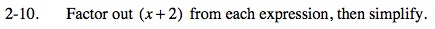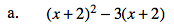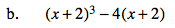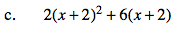### Home > AC > Chapter 13 > Lesson 13.PSF1-S > Problem2-10

2-10.
1. Factor out (x + 2) from each expression, then simplify. Homework Help ✎

1. (x + 2)2 − 3(x + 2)

2. (x + 2)3 − 4(x + 2)

3. 2(x + 2)2 + 6(x + 2)